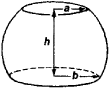# Spherical Segment of Two Bases

## Spherical Segment of Two Bases

a part of a sphere bounded by two parallel planes intersecting the sphere (see Figure 1). The volume of such a solid is V = πh(3a2 + 3b2 + h2)/6,Figure 1

and the area of the solid’s curved surface, which is called a zone, is S = 2πRh; here, R is the radius of the sphere, h is the distance between the planes of the bases, and a and b are the radii of the bases.

Site: Follow: Share:
Open / Close